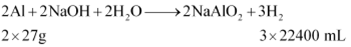# The drain cleaner, Drainex contains small bits of aluminium which react

The drain cleaner, Drainex contains small bits of aluminum which react with caustic soda to produce hydrogen. What volume of hydrogen at 20 degree Celsius and one bar will be released when 0.15g of aluminum reacts?

The reaction of aluminium with caustic soda can be shown as:Therefore volume of hydrogen at STP released when 0.15g of Al reacts
V1 =0.15 x 3 x 22.4 /54 = 187ml
Now P1 = 1 bar,
P2 = 1 bar
T1 = 273 K
T2 = 20 + 273 = 293 K
V1 = 187 ml
V2 = x
When pressure is held constant, then
V2 = P1 V1 T2 / P2 T1
OR
x = 1 × 187 × 293 / 0.987 × 273
= 201 ml
Therefore, 201 mL of dihydrogen will be released.